## 3.8.3 Application to Facility Location and Districting

One could apply the ideas of spatial Poisson processes to a problem of facility location and districting of a city. Suppose that demands are distributed uniformly throughout the plane and suppose that travel distance is right-angle. We can consider two applications: (1) for each service request, a response unit is dispatched from the nearest facility (the service system need not be an emergency service; for instance, it could be a social service agency whose personnel make home visits); and (2) the individual requiring service travels to the nearest facility (e.g., hospital, library, "little city hall," police district station house). Each district about a facility would consist of all points closer to that facility than to any other.
As an agency administrator, you want to get some idea of the potential benefits (in terms of mean travel distance reduction) of a study to optimally locate service facilities.

Use of Poisson model to generate "upper bound." At one extreme, you could ask: What are the response distance characteristics of the system if facilities are distributed at random? We can answer this question by assuming that facilities are distributed as a homogeneous spatial Poisson process. This corresponds to a totally unplanned system (in terms of districting) in which the facility locations could be viewed as occurring from "throwing darts blindfolded" at a map of the city. That is, given n facilities in any particular region, their locations would be independently, uniformly distributed over the region (following the "unordered arrival times" argument of Chapter 2 for a time Poisson process).
For a right-angle distance metric, a random distribution of facilities may yield a city-wide districting as shown in Figure 3.33. Using the result of Example 16, the mean travel distance is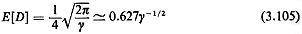where y is the average density of facilities.

Lower bound. To achieve minimal mean travel distance, the facilities should be positioned in a regular lattice, as shown in Figure 3.34. This makes intuitive sense since a diamond gives the set of points within a given distance of its center, when right-angle distance is used (analogous to a circle for Euclidean distance), so diamonds can be used to partition a city into districts of equal coverage, where coverage of a district is measured by maximum possible distance from its facility.
We prove the desired result regarding E[D] in two steps: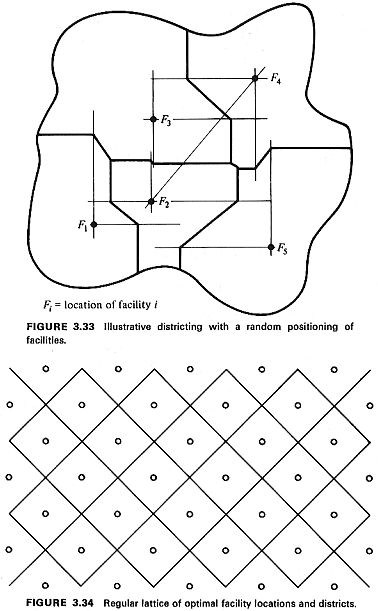STEP 1: Given that a facility's district must contain an area A, a square district rotated at 45°, centered at the facility's position, results in minimum mean travel distance (E[D]).

Proof: (Contradiction, using perturbation method.) Suppose there is some redesign of the rotated square that results in lower mean travel distance. Then the new district can be constructed by taking a set of points B1, of areaout of the rotated square and adding a set of points B2. of area c from outside the square. The situation is shown in Figure 3.35. Then, in the redesigned district, the new mean travel distance is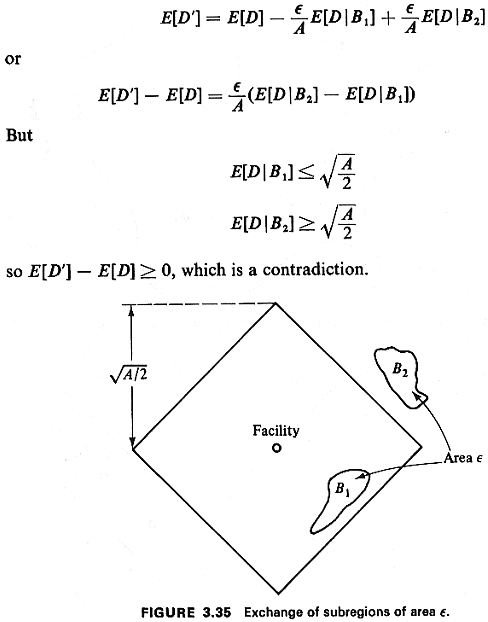STEP 2: Given that we have N square districts, each rotated at 45° and centered at the respective facility's position, and given that the total area of the N districts must equal NA, minimal mean travel time is obtained by setting the area of each district equal to A.

Proof: For a random service request located in one of the N districts, assuming that district i has area Ai, the mean travel distance is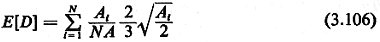(Why?) The idea is to minimize (3.106) subject to the total area constraint,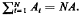. This is a straightforward problem of constrained optimization. Using Lagrange multipliers, one finds that the minimal E[D] is found by setting Ai=A (all i). Finally (and fortunately!), equal-sized square districts rotated at 45° fit into the lattice shown in Figure 3.34. For this lattice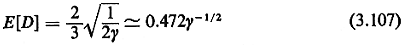Comparing this to the mean travel distance with randomly positioned facilities, we have the somewhat surprising result that optimal positioning (and districting) reduces mean travel distance over that obtained by random positioning by only about 25 percent. What are the policy implications of this result?